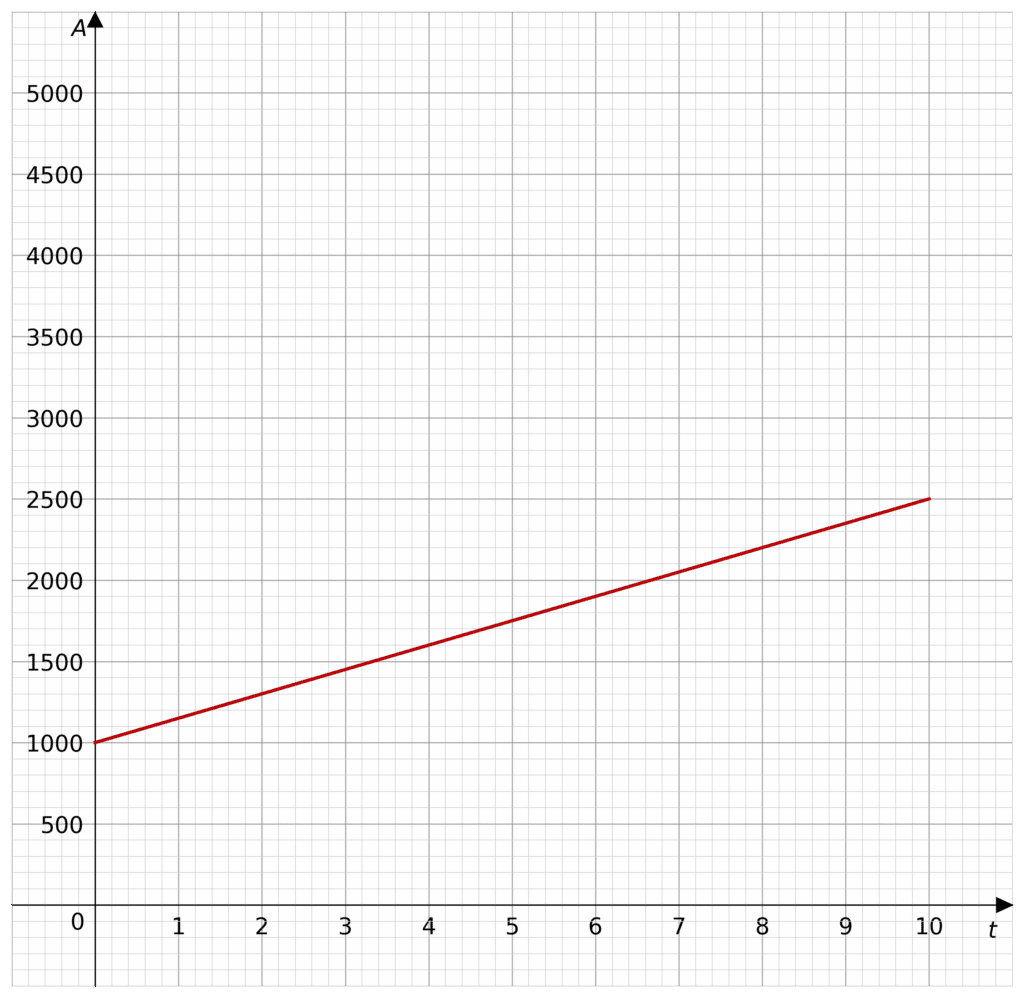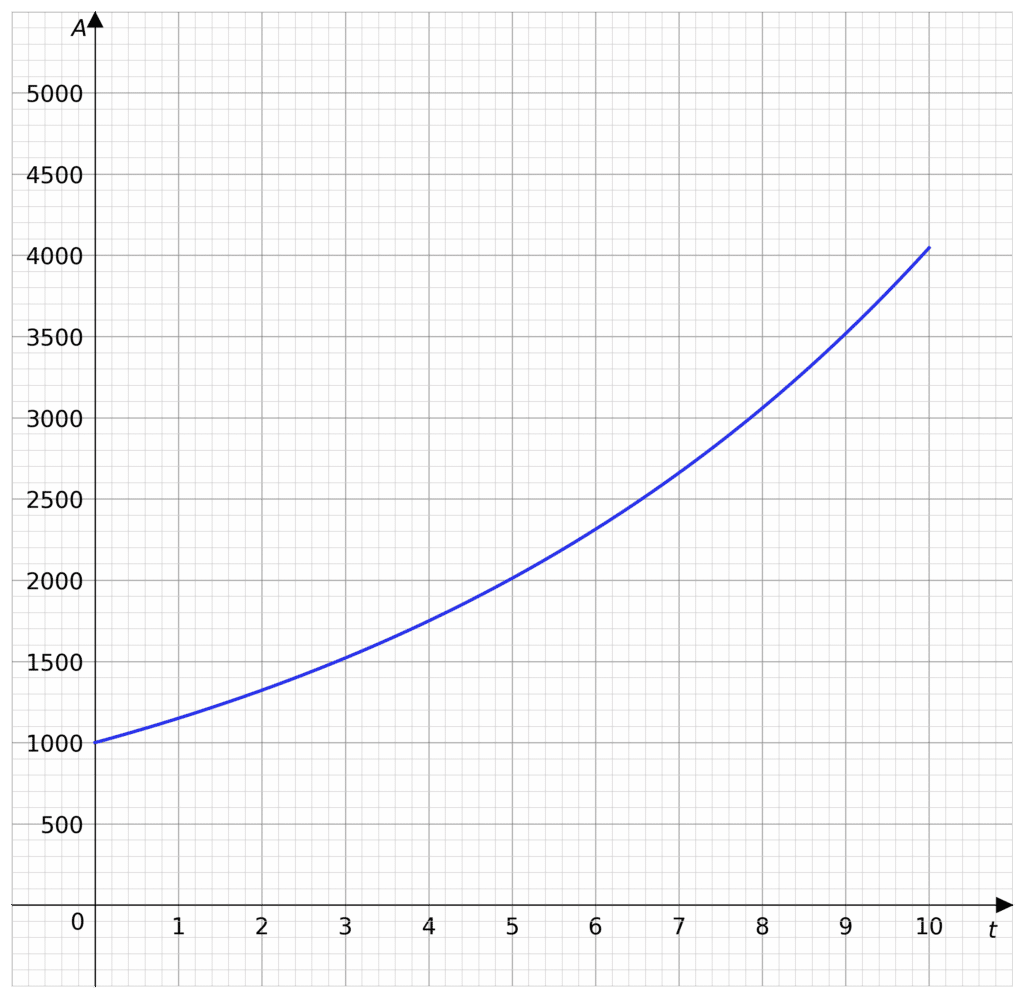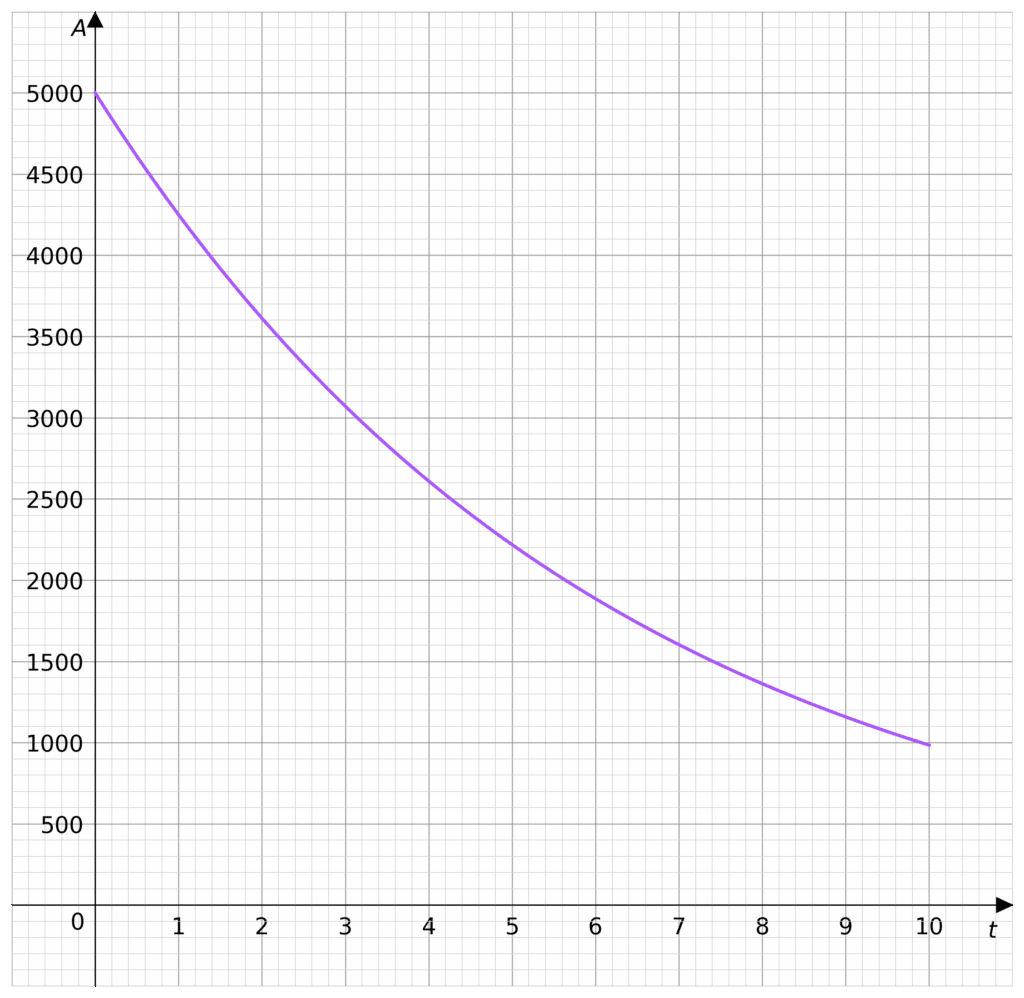# Growth and Decay

GCSELevel 4-5Level 6-7OCR

## Growth and Decay

There are two types of interest we shall look at

• Simple interest, where the interested is calculated against the initial investment
• Compound interest, where the interest calculated on the initial investment and the interest already earned. As you earn interest on the interest, it compounds.

We shall also look at depreciation, where the value decreases by a percentage of itself.

Level 4-5GCSEOCR

## Simple InterestSimple interest takes an initial investment, sometimes called the principal investment, $P$, and increases it by an interest rate, $r$, every increment of time, $t$. This increment of time is usually per year, but could be per month, or per quarter. So $t$ is the time in years for our sake.

Remember if the interest rate is $5\%$ then $5\%$ of $P$ is

$0.05 \times P$

So to increase by $5\%$

$P+0.05P$

So the value of $r=0.05$, and if we want to increase by $5\%$ of $P$ every year then

$P+rPt$

where $t$ is the number of years. So the formula for simple interest is

$A= P+rPt$

or

$A=P(1+rt)$

where $A$ is the final amount. Remember $r$ is as a decimal, not a percentage.

The example picture is when $P=1000$ and the interest rate is $15\%$ under simple interest.

Level 4-5GCSEOCR

## Year-on-Year Compound Interest

Sometimes the interest rate (or depreciation rate) does not stay the same year-on-year. For example, if a classic car is worth $£20,000$ when it is bought by a collector, then if it appreciates in value, the rate may change year upon year. If the rate of appreciation is $10\%, 20\%$ and $15\%$ after $1$, $2$ and $3$ years respectively, then we can calculate the value of the car after each year.

One year after purchasing the car, it will have a value of

$£20,000 \times (1+0.1) = £22,000$

after two years,

$£22,000 \times (1+0.2) = £26,400$

and after three years,

$£26,400 \times (1+0.15) = £30,360$

We can observe that unlike in simple interest, the value appreciates on how much the car was worth at the end of the previous year, not by how much it was worth at the start. This is why we call this compound interest, because the amount of interest received each year is not fixed like in simple interest, but may compound on itself, earning interest on the interest.

Level 4-5GCSEOCR

## Compound InterestCompound interest takes the principal investment, $P$ and increases it by the interest rate, $r$. Then, the next time the interest is added, the interest is calculated on the principal investment and the added interest.

So, let’s say the interest rate is $5\%$ again so $r=0.05$, added once a year. Then you end the first year with

$P(1+0.05) = 1.05P$

Now for the second year, you calculate the added interest form $1.05P$, instead of $P$. So for year $2$ we find $5%$ of $1.05P$

$1.05P\times(1+0.05)= P \times (1.05)^2$

Recalling $1.05 = 1+r$, then for year two

$P\times (1+r)^2$

Similarly for year $3$

$P\times (1+r)^3$

So the formula for compound interest, where $A$ is the total amount after $t$ years is

$A=P(1+r)^t$

The example picture is of when $P = 1000$ and the interest rate of $15\%$ under compound interest.

Level 6-7GCSEOCR

## DecayDecay in financial terms is called depreciation. Decay is when an amount is reduced by a percentage of itself every increment of time. Depreciation is when the value of something decays. Similarly to compound interest, depreciation is compounded.

If an asset (like a car or a stock portfolio) has initial value $P$, and it decreases in value by the depreciation rate of $5\%$, then we calculate that by

$P\times (1-0.05)$

So if it depreciates by depreciation rate $r$, every year, then let $t$ be the time in years, and $A$ be the final amount.

$A=P(1-r)^t$

The example picture is when $P=5000$, and it is depreciating at a rate of $15\%$

Level 6-7GCSEOCR
Level 4-5GCSEOCR

## Example 1: Simple Interest

A loan has simple interest on it. For every month the money is not paid off, $8\%$ of the amount borrowed is added to the total debt. If $£1000$ is borrowed, how much is owed after $4$ months?

[3 marks]

Firstly we call the amount borrowed $P=1000$, the interest rate is $r=0.08$ and $t=4$, so let the total debt be $£A$.

Substituting these into the simple interest equation we get

$A= P(1+rt)$
$A=1000 \times (1+0.08 \times 4)$
$A=1000(1.32)$
$A=1320$

So the total debt is $£1320$

Level 4-5GCSEOCR

## Example 2: Year-on-Year Compound Interest

A car is purchased new for $£25,000$.

This car depreciates in value by $20\%, 15\%$ and $9\%$ after $1, 2$ and $3$ years, respectively.

Calculate the value of the car after $3$ years.

[3 marks]

First, we must work out the value of the car after $1$ year:

$\text{Value} = £25,000 \times (1-0.2) = £20,000$

After $2$ years:

$\text{Value} = £20,000 \times (1-0.15) = £17,000$

After $3$ years:

$\text{Value} = £17,000 \times (1-0.09) = £15,470$

Hence the value of the car after $3$ years shall be $£15,470$

Level 4-5GCSEOCR

## Example 3: Compound Interest

Ethan invests $£156,250$ for $3$ years at $4\%$ compound interest.

Work out the value of this investment after $3$ years. Give your answer to the nearest pound.

[3 marks]

Firstly we all the amount invested $P=156,250$, the interest rate is $r=0.04$ and $t=3$, so let the total value be $£A$.

Substituting these into the compound interest equation we get

$A=P(1+r)^t$
$A=156,250 \times (1+0.04)^3$
$A=156,250(1.04)^3$
$A=175,760$

So the total value after three years is $£175,760$

Level 6-7GCSEOCR

## Growth and Decay Example Questions

Bank $1$: after four years, $P=4000$, $t=4$

$A=4000\times(1+0.04\times 4)$
$A=4000(1.16)$
$A = £4640$

Bank $2$: After four years, $P=4200$, $t=4$

$A=4200\times (1+0.02\times 4)$
$A=4200(1.08)$
$A=£4532$

So for Sarah, Bank $1$ will make her more money.

a) The value of the house appreciates by $8%$ after one year

$\text{Value} = £200,000 \times (1+0.08) = £216,000$

b) After $2$ years:

$\text{Value} = £216,000 \times (1+0.05) = £226,800$

After $3$ years:

$\text{Value} = £226,800 \times (1+0.03) = £233,604$

After $3$ years the house is worth $£233,604$, so the increase in value is

$233,604 - 200,000 = £33,604$

Let the value of the fund be $£A =£12,000$, the initial investment be $£P=£12,000$ and let the interest rate be $r=0.1$

Substituting into the compound interest formula we get

$17,569.2=12,000(1+0.1)^n$
$17,569.2=12,000(1.1)^n$

$\dfrac{17,569.2}{12,000} = 1.1^n$

$1.4641=1.1^n$

So now we can raise $1.1$ to various powers until we find $n$

$1.1^2=1.21$
$1.1^3=1.331$
$1.1^4=1.4641$

So $n=4$

a) If the value new is $P$ and the interest rate is $r=0.12$, then $60\%$ of the cars original value is $0.6P$, so using the depreciation formula we get

$A=P(1-0.12)^t$

Where $t$ is the time in years and $A$ is the total value of the car. So when the value drops below $60\%$ for the first time

$P(0.88)^t<0.6P$
$0.88^t<0.6$

So now we can test values of $t$.

$0.88^2=0.7744$
$0.88^3=0.681472$
$0.88^4=0.59969536 <0.6$

So year $4$ will be the year the cars value drops below $60\%$ for the first time.

b) Using the formula with $P=13,500$ and $t=10$ we get

$A=13,500(1-0.12)^{10}$
$A=13,500(0.88)^{10}$
$A=3759.76317...$

So the value of the car after $10$ years is $£3760$ to the nearest pound.

## You May Also Like...### MME Learning Portal

Online exams, practice questions and revision videos for every GCSE level 9-1 topic! No fees, no trial period, just totally free access to the UK’s best GCSE maths revision platform.

£0.00